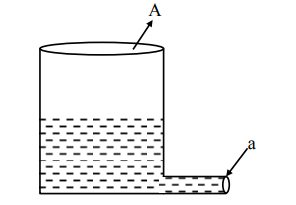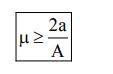# A light cylindrical vessel is kept on a horizontal surface.Question:

A light cylindrical vessel is kept on a horizontal surface. Area of base is A. A hole of crosssectional area 'a' is made just at its bottom side. The minimum coefficient of friction necessary to prevent sliding the vessel due to the impact force of the emerging liquid is (a < < A) :1. $\frac{\mathrm{A}}{2 \mathrm{a}}$

2. None of these

3. $\frac{2 a}{A}$

4. $\frac{\mathrm{a}}{\mathrm{A}}$

Correct Option: , 3

Solution:

For no sliding

$f \geq \rho a v^{2}$

$\mu m g \geq \rho a v^{2}$

$\mu \rho A h g \geq \rho a 2 g h$Option (3)